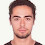Support us .Net Basics C# SQL ASP.NET Aarvi MVC Slides C# Programs Subscribe Download

### Part 4 - LINQ Aggregate Functions

Suggested Videos
Part 1 - What is LINQ
Part 2 - Writing LINQ Queries
Part 3 - Extension Methods

LINQ Standard Query Operators also called as LINQ extension methods can be broadly classified into the following categories
Aggregate Operators
Grouping Operators
Restriction Operators
Projection Operators
Set Operators
Partitioning Operators
Conversion Operators
Element Operators
Ordering Operators
Generation Operators
Query Execution
Join Operators
Custom Sequence Operators
Quantifiers Operators
Miscellaneous Operators

In this video we will discuss the following LINQ Aggregate Operators
Min
Max
Sum
Count
Average
Aggregate (Next Video)

Example 1:
using System;
using System.Linq;
namespace Demo
{
class Program
{
static void Main()
{
int[] Numbers = { 1, 2, 3, 4, 5, 6, 7, 8, 9, 10 };

int smallestNumber = Numbers.Min();
int smallestEvenNumber = Numbers.Where(n => n % 2 == 0).Min();

int largestNumber = Numbers.Max();
int largestEvenNumber = Numbers.Where(n => n % 2 == 0).Max();

int sumOfAllNumbers = Numbers.Sum();
int sumOfAllEvenNumbers = Numbers.Where(n => n % 2 == 0).Sum();

int countOfAllNumbers = Numbers.Count();
int countOfAllEvenNumbers = Numbers.Where(n => n % 2 == 0).Count();

double averageOfAllNumbers = Numbers.Average();
double averageOfAllEvenNumbers = Numbers.Where(n => n % 2 == 0).Average();

Console.WriteLine("Smallest Number = " + smallestNumber);
Console.WriteLine("Smallest Even Number = " + smallestEvenNumber);

Console.WriteLine("Largest Number = " + largestNumber);
Console.WriteLine("Largest Even Number = " + largestEvenNumber);

Console.WriteLine("Sum of All Numbers = " + sumOfAllNumbers);
Console.WriteLine("Sum of All Even Numbers = " + sumOfAllEvenNumbers);

Console.WriteLine("Count of All Numbers = " + countOfAllNumbers);
Console.WriteLine("Count of All Even Numbers = " + countOfAllEvenNumbers);

Console.WriteLine("Average of All Numbers = " + averageOfAllNumbers);
Console.WriteLine("Average of All Even Numbers = " + averageOfAllEvenNumbers);
}
}
}

Example 2:
using System;
using System.Linq;
namespace Demo
{
class Program
{
static void Main()
{
string[] countries = { "India", "USA", "UK" };

int minCount = countries.Min(x => x.Length);
int maxCount = countries.Max(x => x.Length);

Console.WriteLine
("The shortest country name has {0} characters in its name", minCount);
Console.WriteLine
("The longest country name has {0} characters in its name", maxCount);
}
}
}1.You are awesome sir..Have seen many videos of yours such a excellent teaching

2.hi venkat sir i am very thankful to you for my entire lifetime.. its only because of you i got the job as a .net developer

3.Great vídeo tutorials, it's being very helpful, thank you!

It would be great if you can help share these free resources